Скачать презентацию CHAPTER 17 Money Growth and Inflation Macroeonomics PRINCIPLES

a787a4058b97b275af0505bb9b5326ae.ppt

• Количество слайдов: 52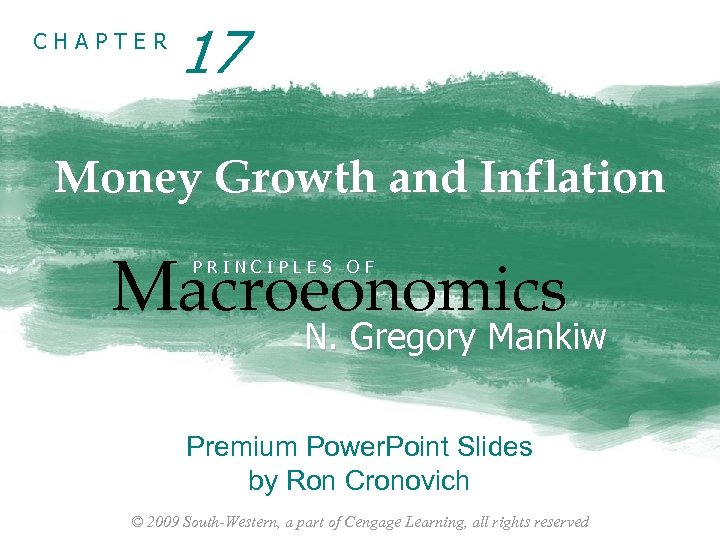CHAPTER 17 Money Growth and Inflation Macroeonomics PRINCIPLES OF N. Gregory Mankiw Premium Power. Point Slides by Ron Cronovich © 2009 South-Western, a part of Cengage Learning, all rights reserved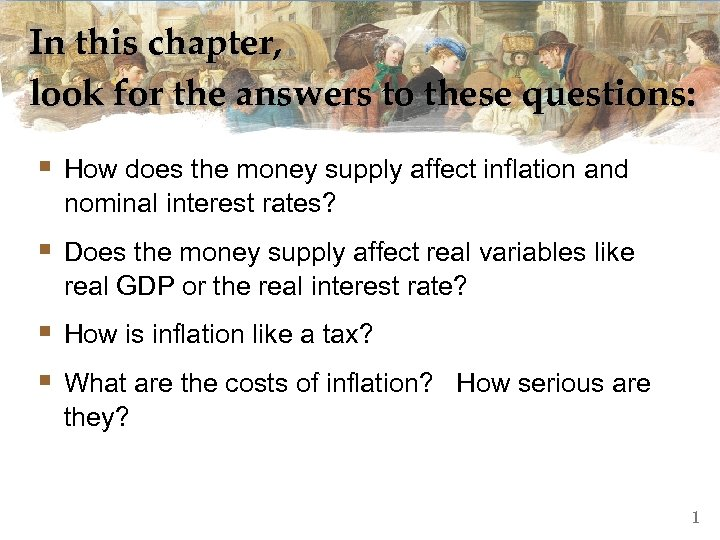In this chapter, look for the answers to these questions: § How does the money supply affect inflation and nominal interest rates? § Does the money supply affect real variables like real GDP or the real interest rate? § How is inflation like a tax? § What are the costs of inflation? How serious are they? 1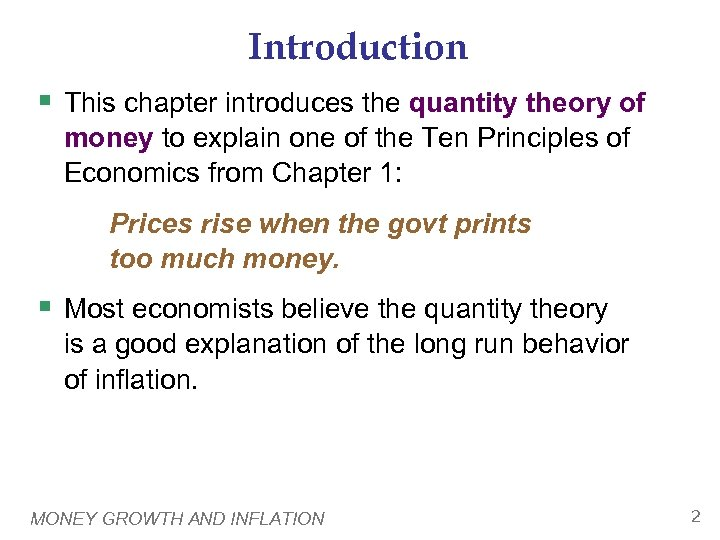Introduction § This chapter introduces the quantity theory of money to explain one of the Ten Principles of Economics from Chapter 1: Prices rise when the govt prints too much money. § Most economists believe the quantity theory is a good explanation of the long run behavior of inflation. MONEY GROWTH AND INFLATION 2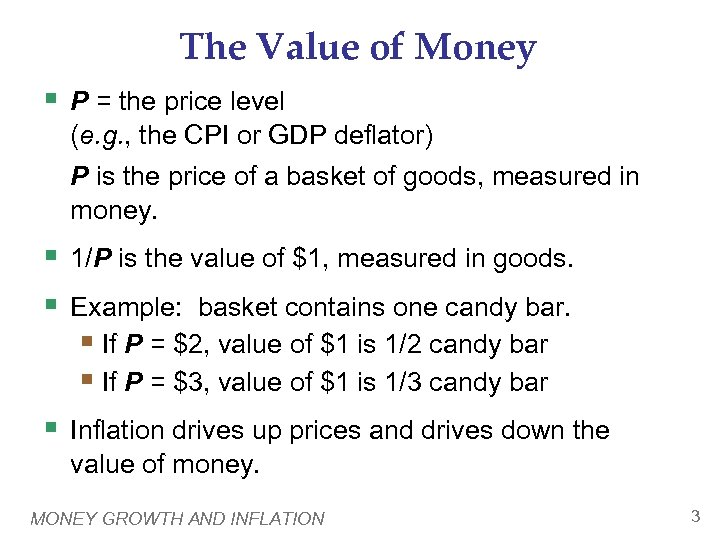The Value of Money § P = the price level (e. g. , the CPI or GDP deflator) P is the price of a basket of goods, measured in money. § 1/P is the value of \$1, measured in goods. § Example: basket contains one candy bar. § If P = \$2, value of \$1 is 1/2 candy bar § If P = \$3, value of \$1 is 1/3 candy bar § Inflation drives up prices and drives down the value of money. MONEY GROWTH AND INFLATION 3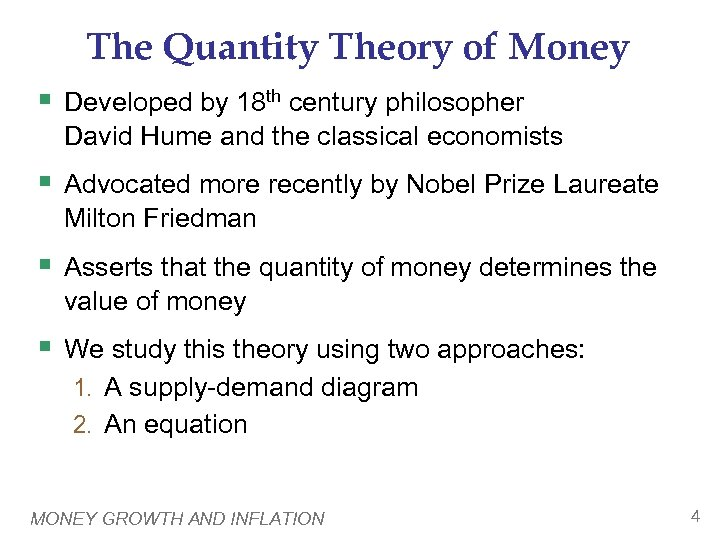The Quantity Theory of Money § Developed by 18 th century philosopher David Hume and the classical economists § Advocated more recently by Nobel Prize Laureate Milton Friedman § Asserts that the quantity of money determines the value of money § We study this theory using two approaches: 1. A supply-demand diagram 2. An equation MONEY GROWTH AND INFLATION 4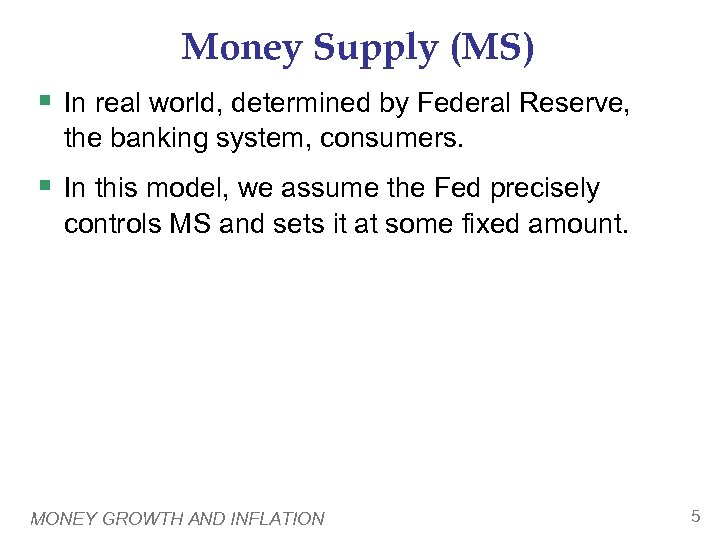Money Supply (MS) § In real world, determined by Federal Reserve, the banking system, consumers. § In this model, we assume the Fed precisely controls MS and sets it at some fixed amount. MONEY GROWTH AND INFLATION 5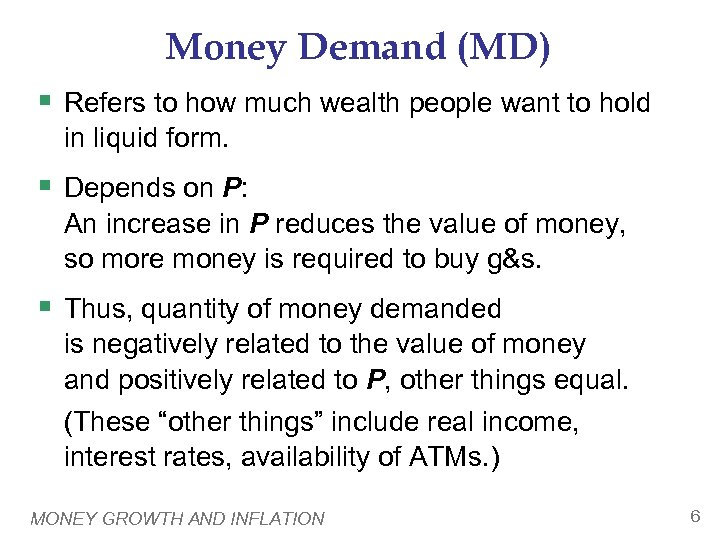Money Demand (MD) § Refers to how much wealth people want to hold in liquid form. § Depends on P: An increase in P reduces the value of money, so more money is required to buy g&s. § Thus, quantity of money demanded is negatively related to the value of money and positively related to P, other things equal. (These “other things” include real income, interest rates, availability of ATMs. ) MONEY GROWTH AND INFLATION 6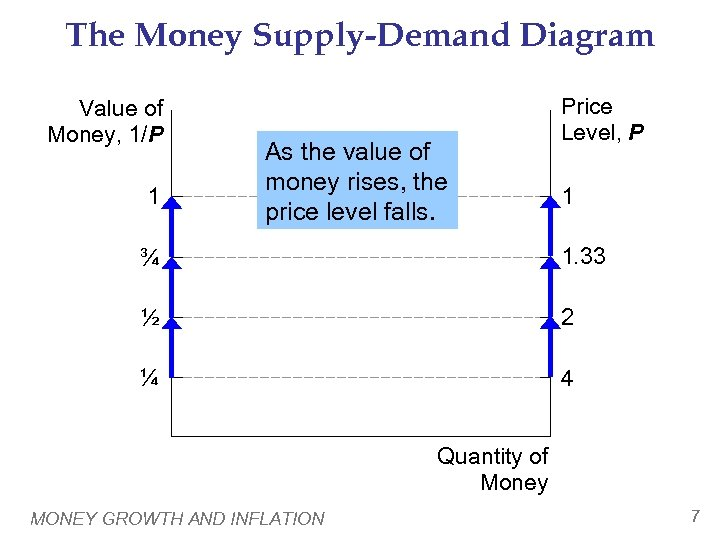The Money Supply-Demand Diagram Value of Money, 1/P 1 As the value of money rises, the price level falls. Price Level, P 1 ¾ 1. 33 ½ 2 ¼ 4 Quantity of Money MONEY GROWTH AND INFLATION 7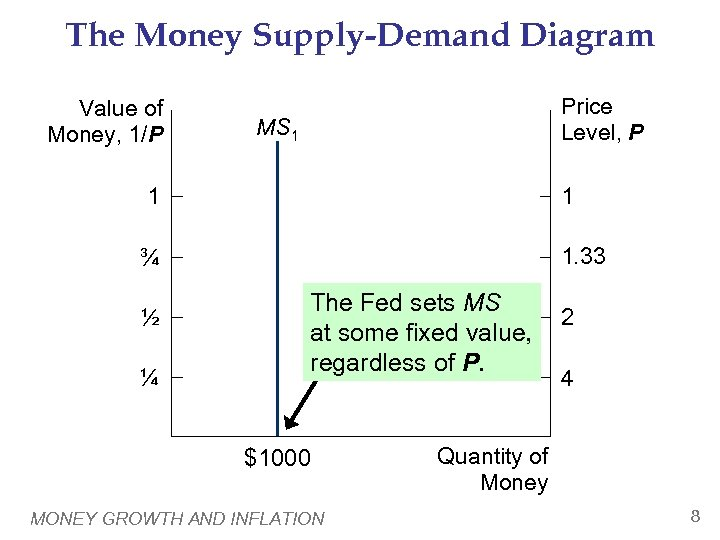The Money Supply-Demand Diagram Value of Money, 1/P Price Level, P MS 1 1 1 ¾ 1. 33 ½ ¼ The Fed sets MS at some fixed value, regardless of P. \$1000 MONEY GROWTH AND INFLATION 2 4 Quantity of Money 8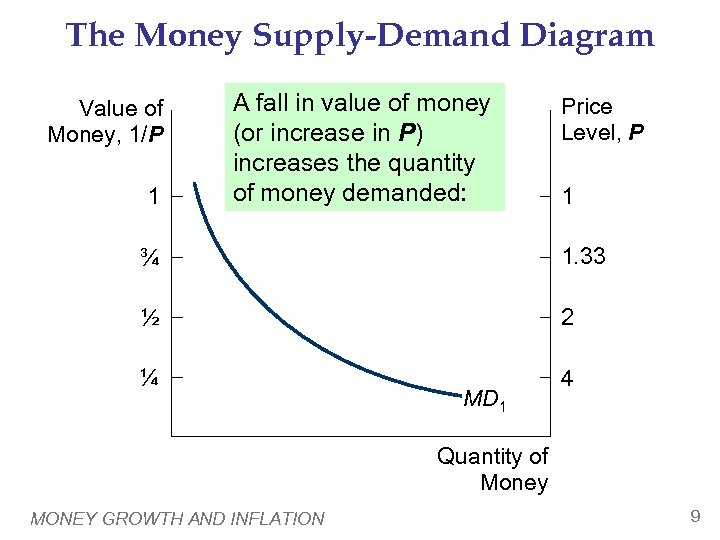The Money Supply-Demand Diagram Value of Money, 1/P 1 A fall in value of money (or increase in P) increases the quantity of money demanded: Price Level, P 1 ¾ 1. 33 ½ 2 ¼ 4 MD 1 Quantity of Money MONEY GROWTH AND INFLATION 9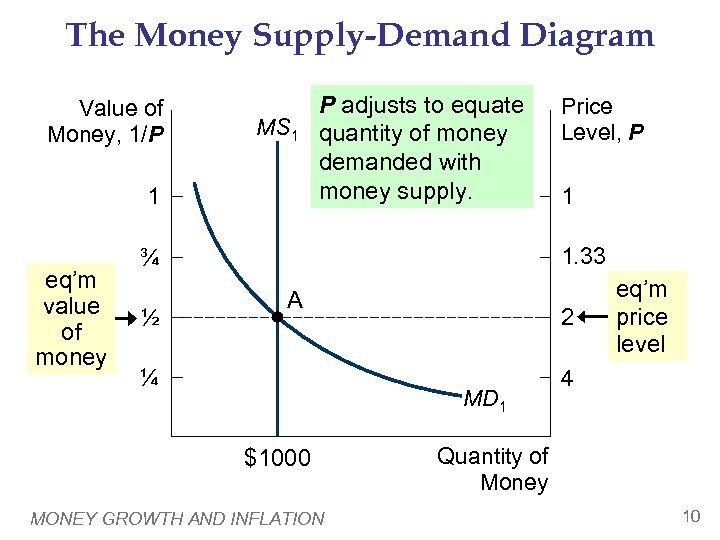The Money Supply-Demand Diagram Value of Money, 1/P MS 1 1 eq’m value of money P adjusts to equate quantity of money demanded with money supply. 1 1. 33 ¾ ½ Price Level, P A ¼ 2 MD 1 \$1000 MONEY GROWTH AND INFLATION eq’m price level 4 Quantity of Money 10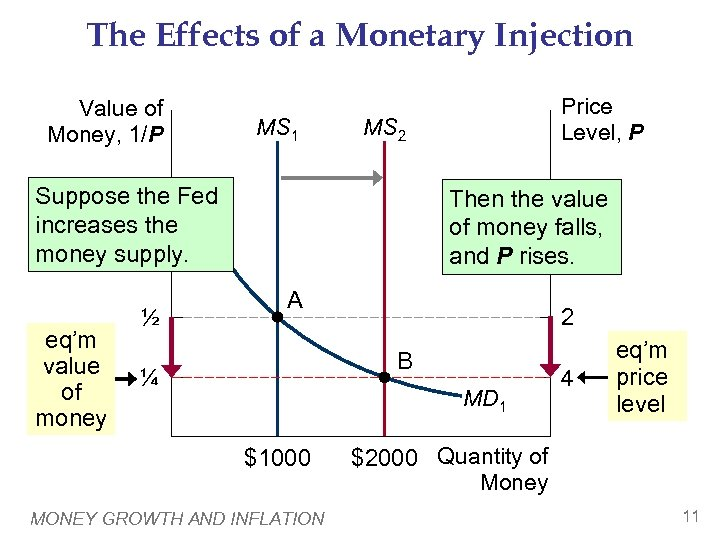The Effects of a Monetary Injection Value of Money, 1/P MS 1 MS 2 1 Suppose the Fed increases the money supply. ¾ eq’m value of money ½ Price Level, P 1 Then the value of money falls, and P rises. 1. 33 A 2 B ¼ MD 1 \$1000 MONEY GROWTH AND INFLATION 4 eq’m price level \$2000 Quantity of Money 11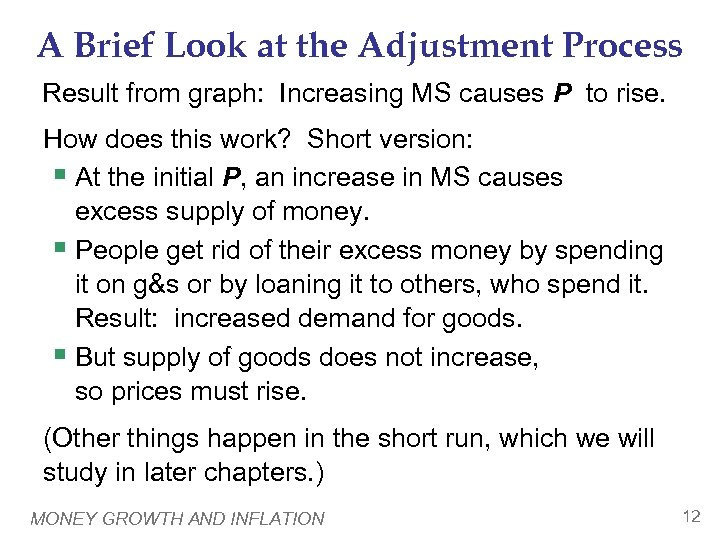A Brief Look at the Adjustment Process Result from graph: Increasing MS causes P to rise. How does this work? Short version: § At the initial P, an increase in MS causes excess supply of money. § People get rid of their excess money by spending it on g&s or by loaning it to others, who spend it. Result: increased demand for goods. § But supply of goods does not increase, so prices must rise. (Other things happen in the short run, which we will study in later chapters. ) MONEY GROWTH AND INFLATION 12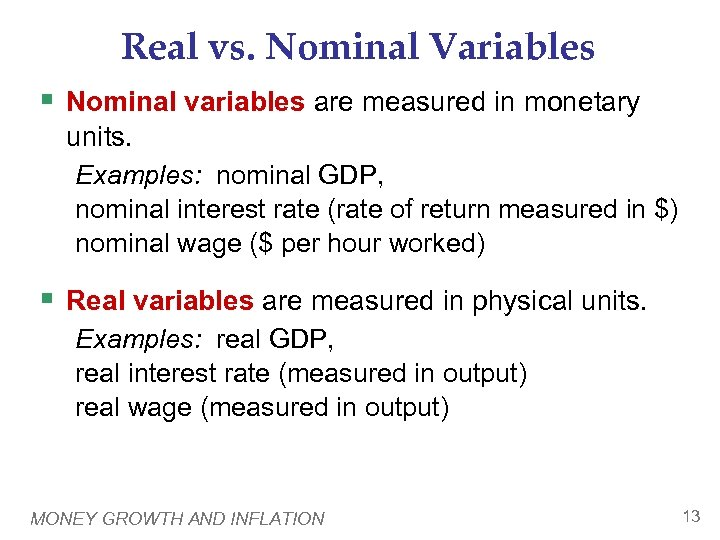Real vs. Nominal Variables § Nominal variables are measured in monetary units. Examples: nominal GDP, nominal interest rate (rate of return measured in \$) nominal wage (\$ per hour worked) § Real variables are measured in physical units. Examples: real GDP, real interest rate (measured in output) real wage (measured in output) MONEY GROWTH AND INFLATION 13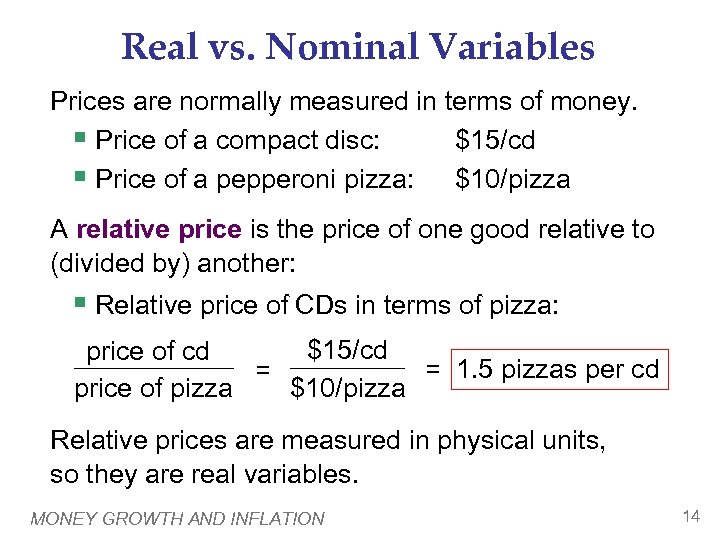Real vs. Nominal Variables Prices are normally measured in terms of money. § Price of a compact disc: \$15/cd § Price of a pepperoni pizza: \$10/pizza A relative price is the price of one good relative to (divided by) another: § Relative price of CDs in terms of pizza: \$15/cd price of cd = 1. 5 pizzas per cd = \$10/pizza price of pizza Relative prices are measured in physical units, so they are real variables. MONEY GROWTH AND INFLATION 14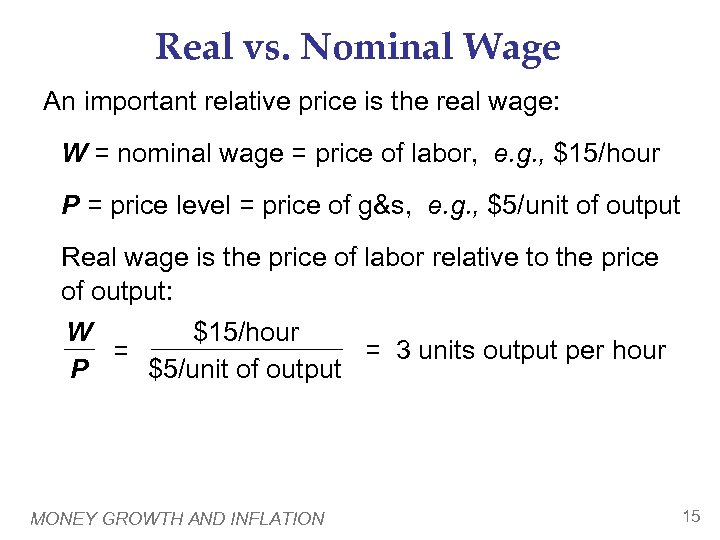Real vs. Nominal Wage An important relative price is the real wage: W = nominal wage = price of labor, e. g. , \$15/hour P = price level = price of g&s, e. g. , \$5/unit of output Real wage is the price of labor relative to the price of output: \$15/hour W = 3 units output per hour = \$5/unit of output P MONEY GROWTH AND INFLATION 15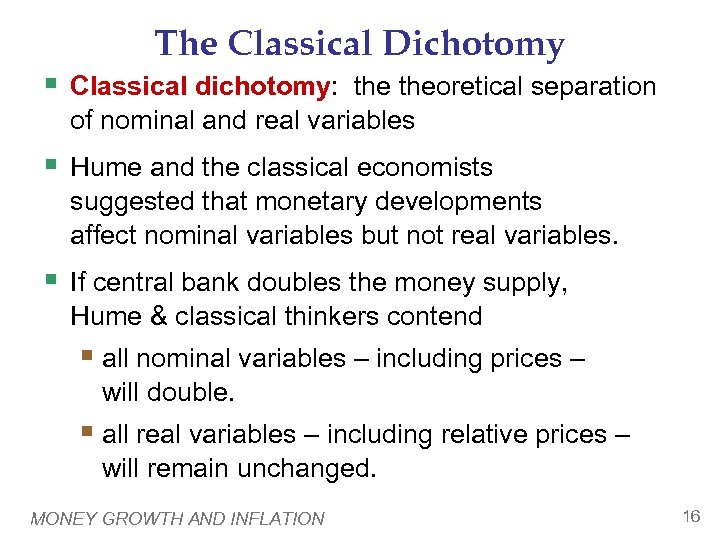The Classical Dichotomy § Classical dichotomy: theoretical separation of nominal and real variables § Hume and the classical economists suggested that monetary developments affect nominal variables but not real variables. § If central bank doubles the money supply, Hume & classical thinkers contend § all nominal variables – including prices – will double. § all real variables – including relative prices – will remain unchanged. MONEY GROWTH AND INFLATION 16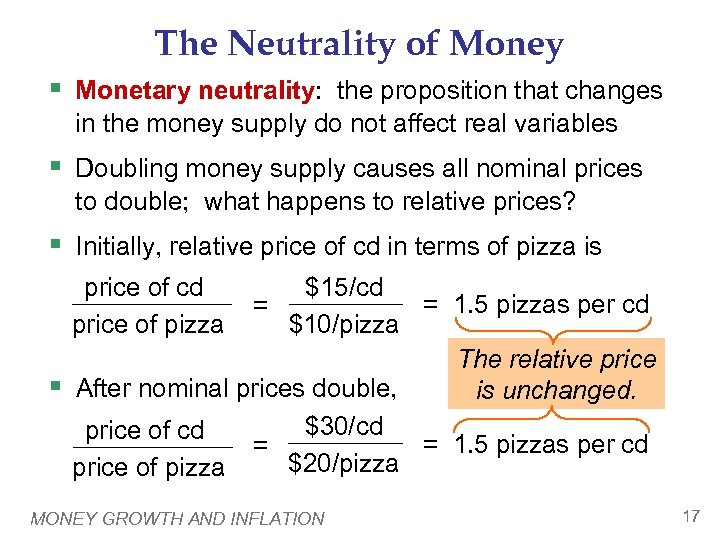The Neutrality of Money § Monetary neutrality: the proposition that changes in the money supply do not affect real variables § Doubling money supply causes all nominal prices to double; what happens to relative prices? § Initially, relative price of cd in terms of pizza is \$15/cd = 1. 5 pizzas per cd = \$10/pizza The relative price After nominal prices double, is unchanged. \$30/cd price of cd = 1. 5 pizzas per cd = \$20/pizza price of cd price of pizza § MONEY GROWTH AND INFLATION 17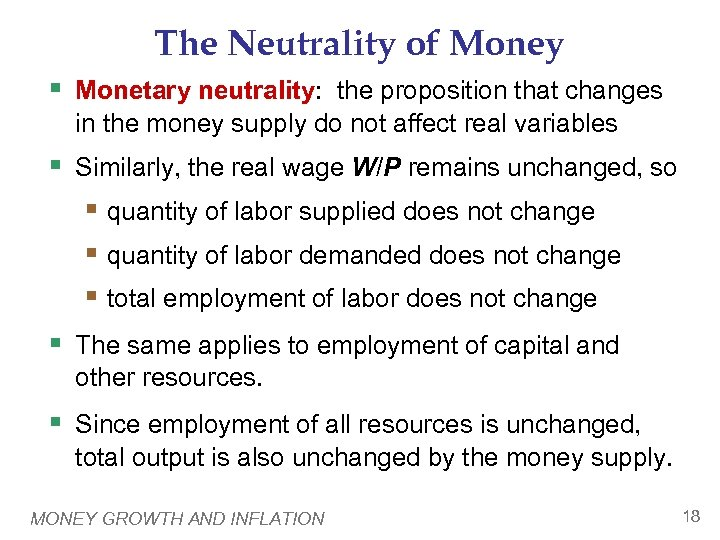The Neutrality of Money § Monetary neutrality: the proposition that changes in the money supply do not affect real variables § Similarly, the real wage W/P remains unchanged, so § quantity of labor supplied does not change § quantity of labor demanded does not change § total employment of labor does not change § The same applies to employment of capital and other resources. § Since employment of all resources is unchanged, total output is also unchanged by the money supply. MONEY GROWTH AND INFLATION 18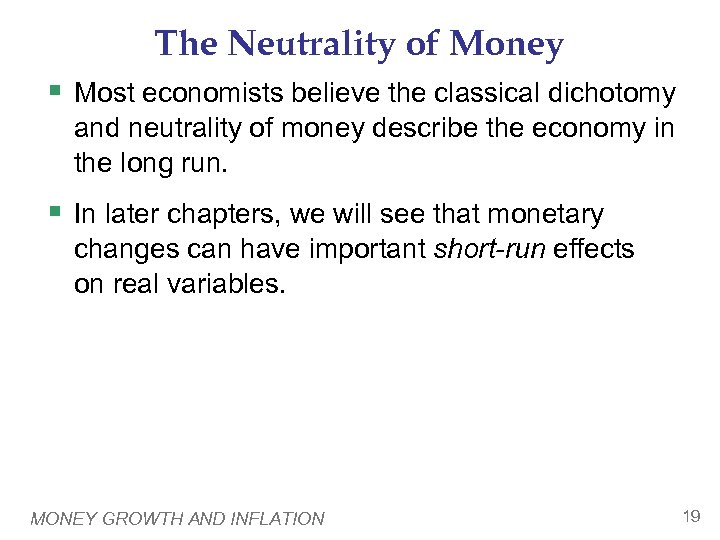The Neutrality of Money § Most economists believe the classical dichotomy and neutrality of money describe the economy in the long run. § In later chapters, we will see that monetary changes can have important short-run effects on real variables. MONEY GROWTH AND INFLATION 19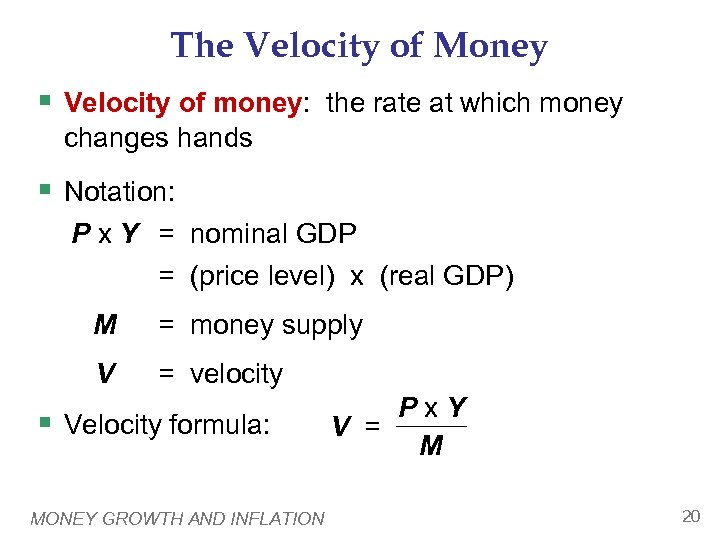The Velocity of Money § Velocity of money: the rate at which money changes hands § Notation: P x Y = nominal GDP = (price level) x (real GDP) M = money supply V = velocity § Velocity formula: MONEY GROWTH AND INFLATION Px. Y V = M 20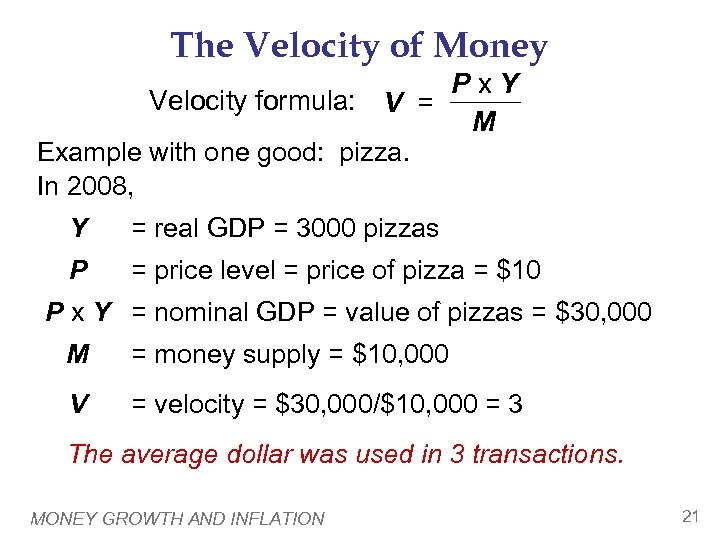The Velocity of Money Px. Y Velocity formula: V = M Example with one good: pizza. In 2008, Y = real GDP = 3000 pizzas P = price level = price of pizza = \$10 P x Y = nominal GDP = value of pizzas = \$30, 000 M = money supply = \$10, 000 V = velocity = \$30, 000/\$10, 000 = 3 The average dollar was used in 3 transactions. MONEY GROWTH AND INFLATION 21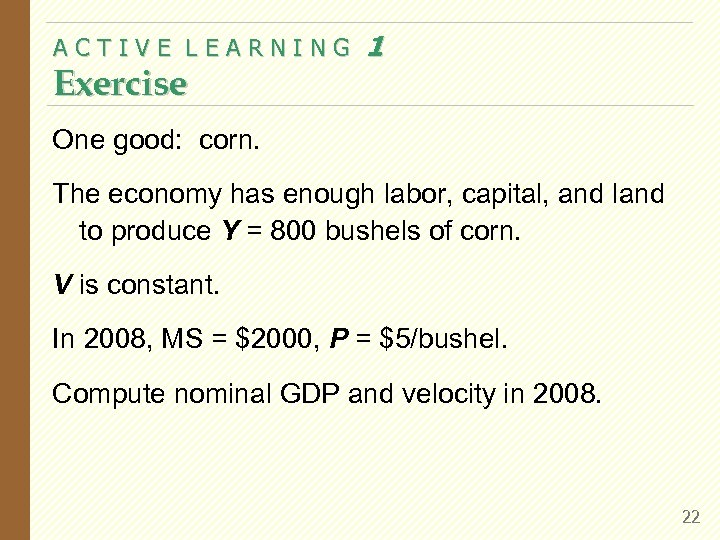ACTIVE LEARNING Exercise 1 One good: corn. The economy has enough labor, capital, and land to produce Y = 800 bushels of corn. V is constant. In 2008, MS = \$2000, P = \$5/bushel. Compute nominal GDP and velocity in 2008. 22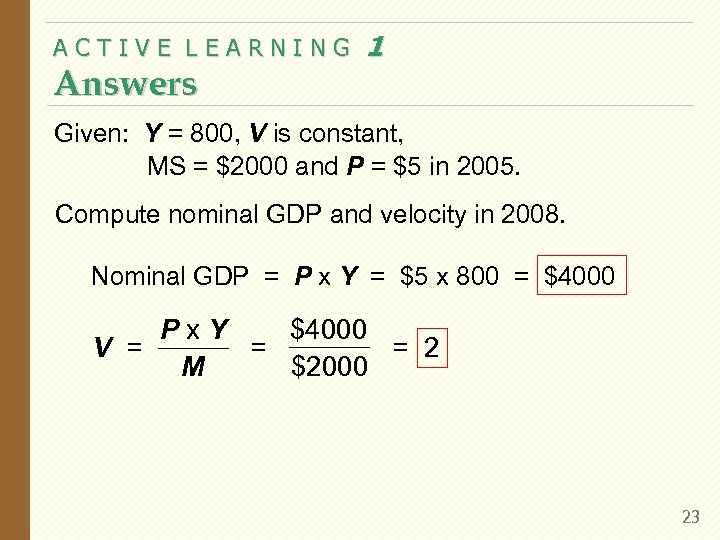ACTIVE LEARNING Answers 1 Given: Y = 800, V is constant, MS = \$2000 and P = \$5 in 2005. Compute nominal GDP and velocity in 2008. Nominal GDP = P x Y = \$5 x 800 = \$4000 Px. Y = 2 = V = \$2000 M 23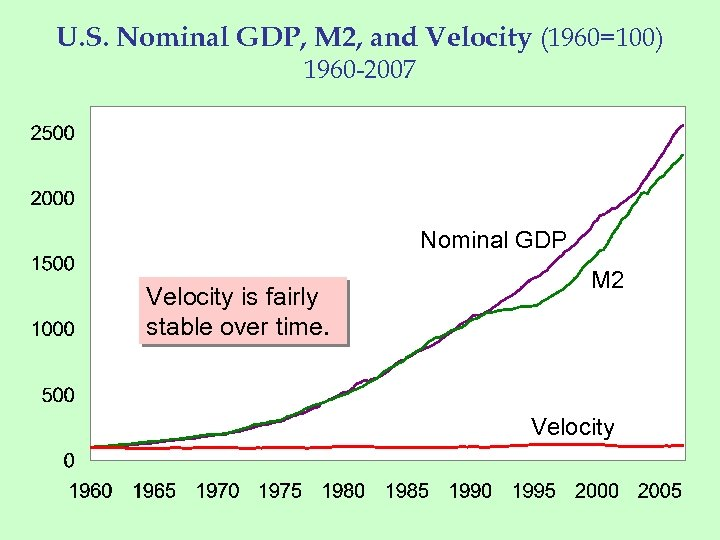U. S. Nominal GDP, M 2, and Velocity (1960=100) 1960 -2007 Nominal GDP Velocity is fairly stable over time. M 2 Velocity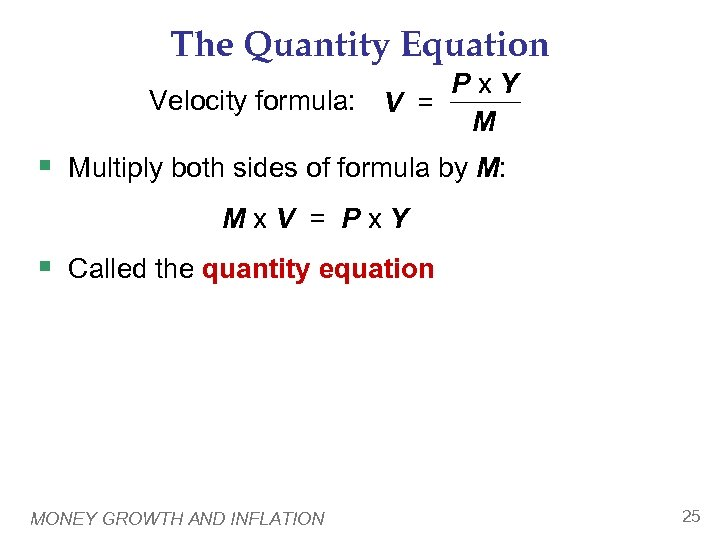The Quantity Equation Px. Y Velocity formula: V = M § Multiply both sides of formula by M: Mx. V = Px. Y § Called the quantity equation MONEY GROWTH AND INFLATION 25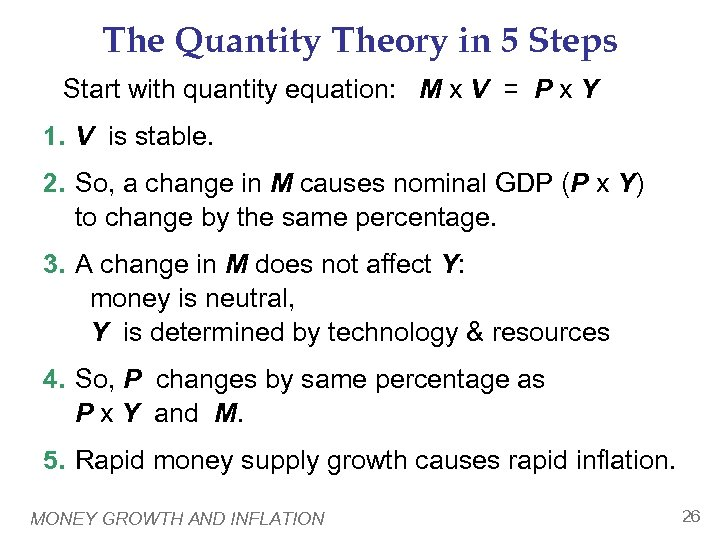The Quantity Theory in 5 Steps Start with quantity equation: M x V = P x Y 1. V is stable. 2. So, a change in M causes nominal GDP (P x Y) to change by the same percentage. 3. A change in M does not affect Y: money is neutral, Y is determined by technology & resources 4. So, P changes by same percentage as P x Y and M. 5. Rapid money supply growth causes rapid inflation. MONEY GROWTH AND INFLATION 26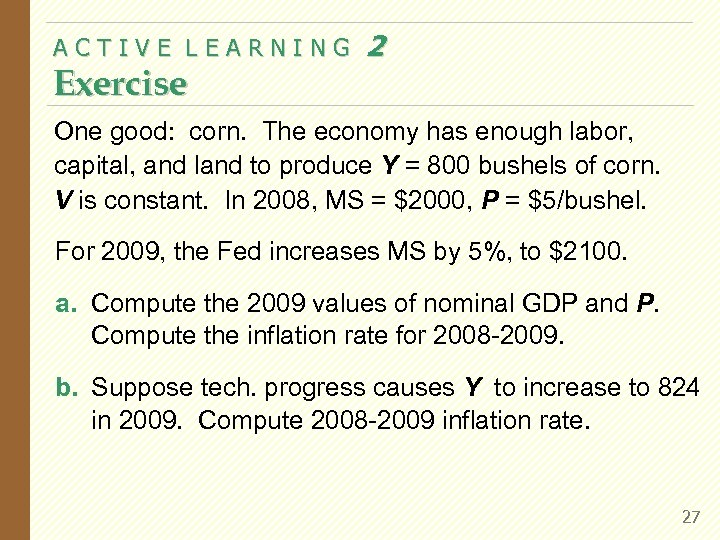ACTIVE LEARNING Exercise 2 One good: corn. The economy has enough labor, capital, and land to produce Y = 800 bushels of corn. V is constant. In 2008, MS = \$2000, P = \$5/bushel. For 2009, the Fed increases MS by 5%, to \$2100. a. Compute the 2009 values of nominal GDP and P. Compute the inflation rate for 2008 -2009. b. Suppose tech. progress causes Y to increase to 824 in 2009. Compute 2008 -2009 inflation rate. 27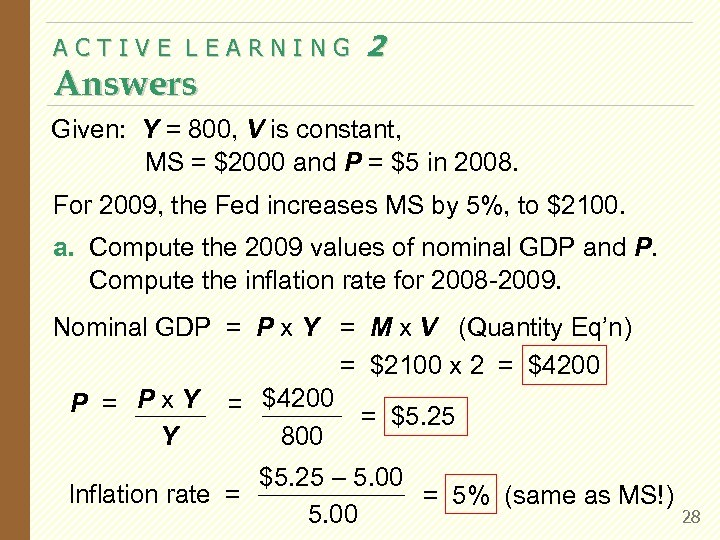ACTIVE LEARNING Answers 2 Given: Y = 800, V is constant, MS = \$2000 and P = \$5 in 2008. For 2009, the Fed increases MS by 5%, to \$2100. a. Compute the 2009 values of nominal GDP and P. Compute the inflation rate for 2008 -2009. Nominal GDP = P x Y = M x V (Quantity Eq’n) = \$2100 x 2 = \$4200 P = P x Y = \$4200 = \$5. 25 800 Y \$5. 25 – 5. 00 Inflation rate = = 5% (same as MS!) 5. 00 28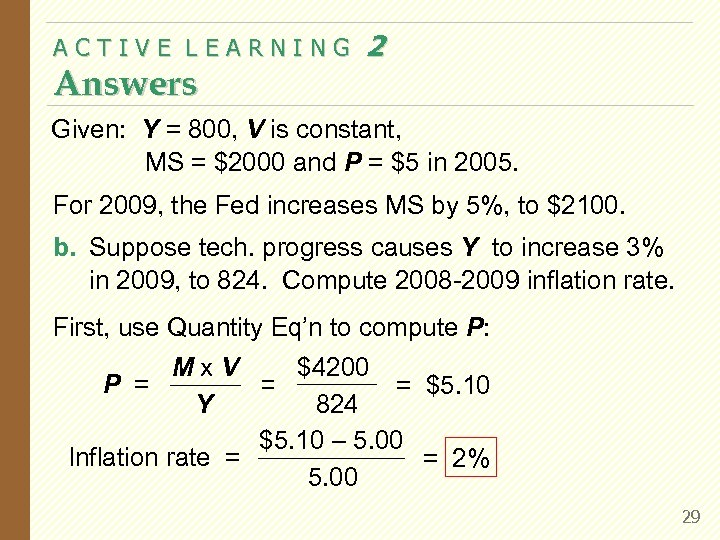ACTIVE LEARNING Answers 2 Given: Y = 800, V is constant, MS = \$2000 and P = \$5 in 2005. For 2009, the Fed increases MS by 5%, to \$2100. b. Suppose tech. progress causes Y to increase 3% in 2009, to 824. Compute 2008 -2009 inflation rate. First, use Quantity Eq’n to compute P: \$4200 Mx. V P = = \$5. 10 = 824 Y \$5. 10 – 5. 00 Inflation rate = = 2% 5. 00 29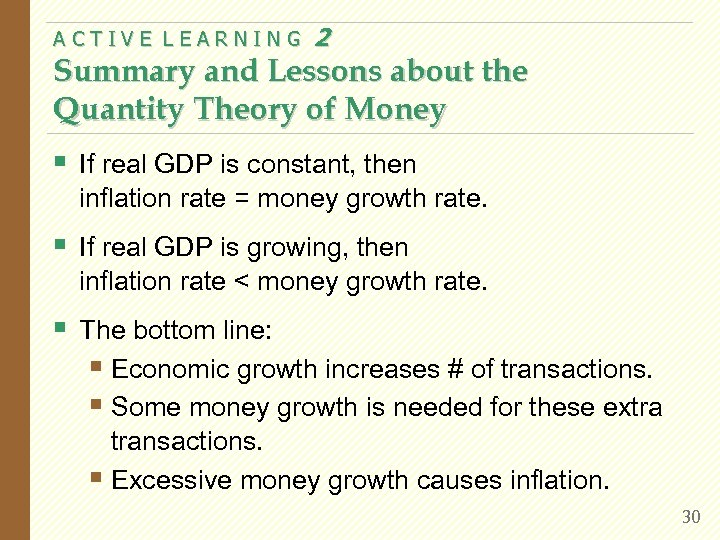ACTIVE LEARNING 2 Summary and Lessons about the Quantity Theory of Money § If real GDP is constant, then inflation rate = money growth rate. § If real GDP is growing, then inflation rate < money growth rate. § The bottom line: § Economic growth increases # of transactions. § Some money growth is needed for these extra transactions. § Excessive money growth causes inflation. 30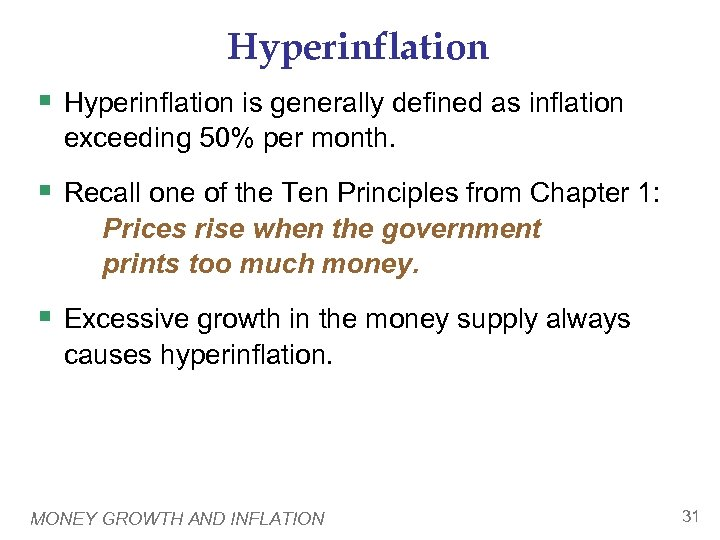Hyperinflation § Hyperinflation is generally defined as inflation exceeding 50% per month. § Recall one of the Ten Principles from Chapter 1: Prices rise when the government prints too much money. § Excessive growth in the money supply always causes hyperinflation. MONEY GROWTH AND INFLATION 31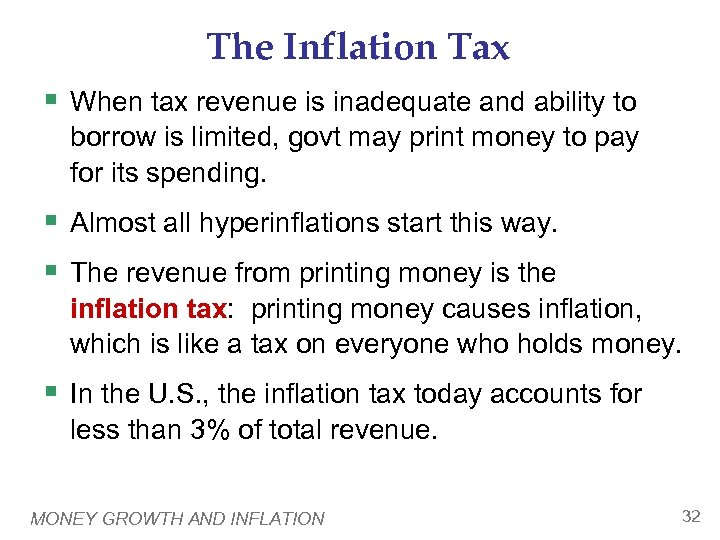The Inflation Tax § When tax revenue is inadequate and ability to borrow is limited, govt may print money to pay for its spending. § Almost all hyperinflations start this way. § The revenue from printing money is the inflation tax: printing money causes inflation, which is like a tax on everyone who holds money. § In the U. S. , the inflation tax today accounts for less than 3% of total revenue. MONEY GROWTH AND INFLATION 32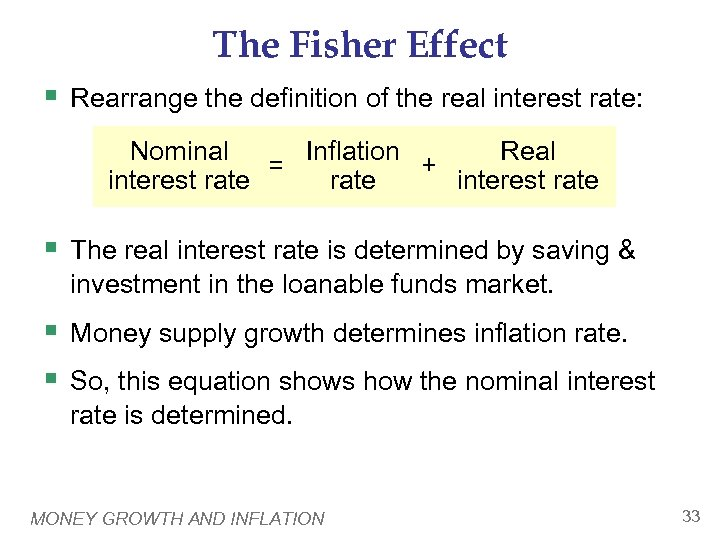The Fisher Effect § Rearrange the definition of the real interest rate: Nominal Real Inflation + = interest rate § The real interest rate is determined by saving & investment in the loanable funds market. § Money supply growth determines inflation rate. § So, this equation shows how the nominal interest rate is determined. MONEY GROWTH AND INFLATION 33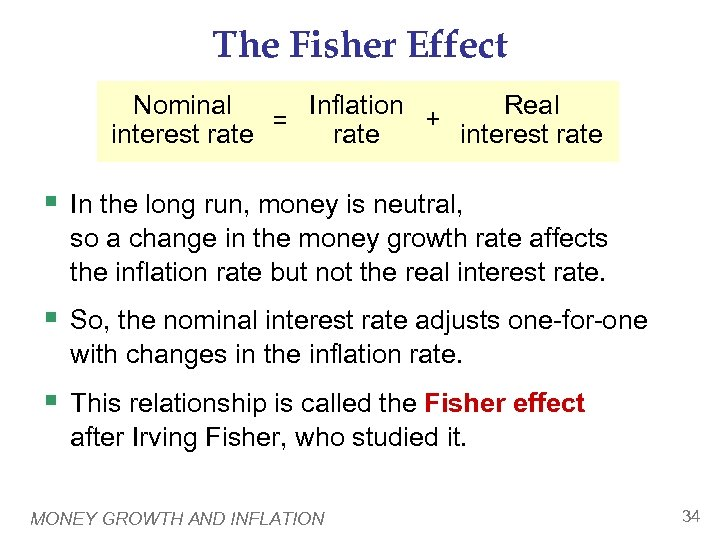The Fisher Effect Nominal Real Inflation + = interest rate § In the long run, money is neutral, so a change in the money growth rate affects the inflation rate but not the real interest rate. § So, the nominal interest rate adjusts one-for-one with changes in the inflation rate. § This relationship is called the Fisher effect after Irving Fisher, who studied it. MONEY GROWTH AND INFLATION 34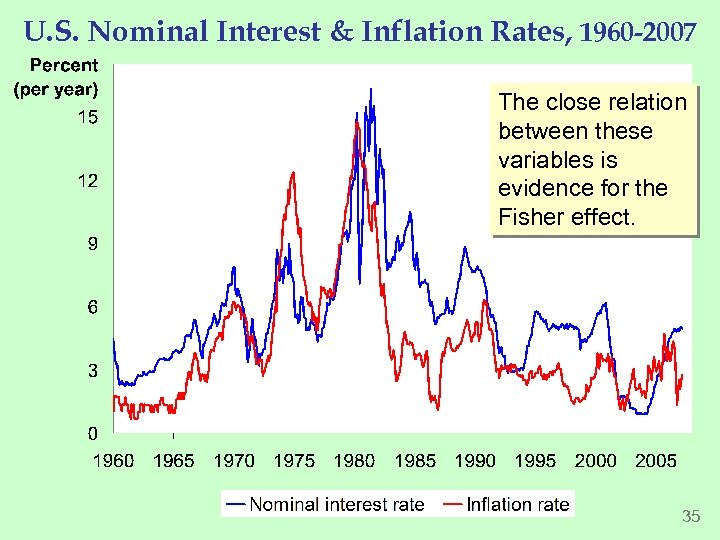U. S. Nominal Interest & Inflation Rates, 1960 -2007 The close relation between these variables is evidence for the Fisher effect. 35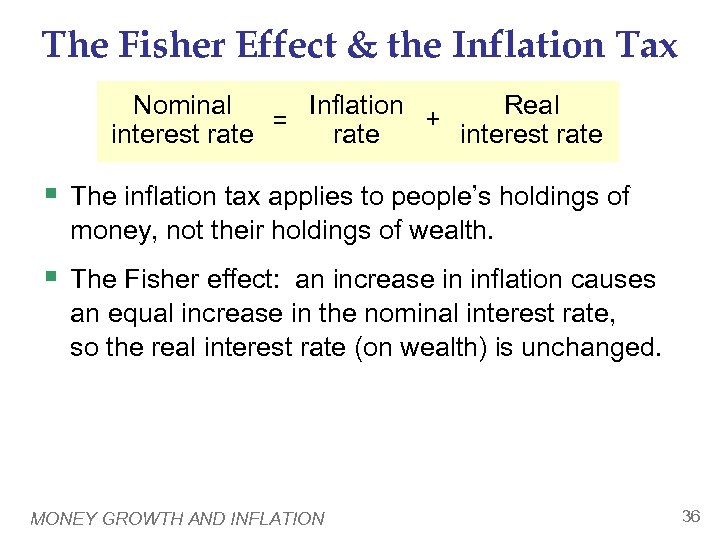The Fisher Effect & the Inflation Tax Nominal Real Inflation + = interest rate § The inflation tax applies to people’s holdings of money, not their holdings of wealth. § The Fisher effect: an increase in inflation causes an equal increase in the nominal interest rate, so the real interest rate (on wealth) is unchanged. MONEY GROWTH AND INFLATION 36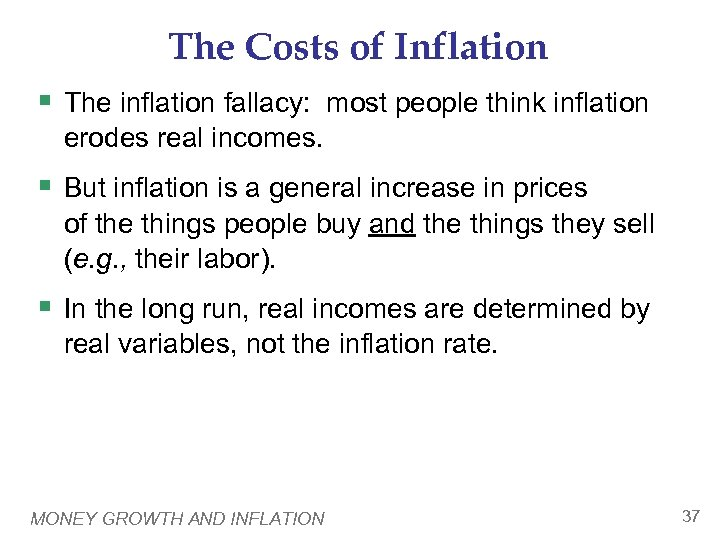The Costs of Inflation § The inflation fallacy: most people think inflation erodes real incomes. § But inflation is a general increase in prices of the things people buy and the things they sell (e. g. , their labor). § In the long run, real incomes are determined by real variables, not the inflation rate. MONEY GROWTH AND INFLATION 37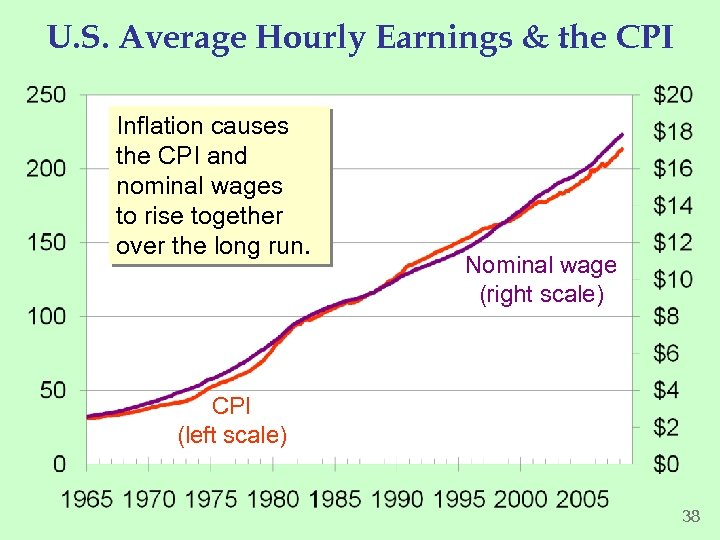U. S. Average Hourly Earnings & the CPI Inflation causes the CPI and nominal wages to rise together over the long run. Nominal wage (right scale) CPI (left scale) 38The Costs of Inflation § Shoeleather costs: the resources wasted when inflation encourages people to reduce their money holdings § Includes the time and transactions costs of more frequent bank withdrawals § Menu costs: the costs of changing prices § Printing new menus, mailing new catalogs, etc. MONEY GROWTH AND INFLATION 39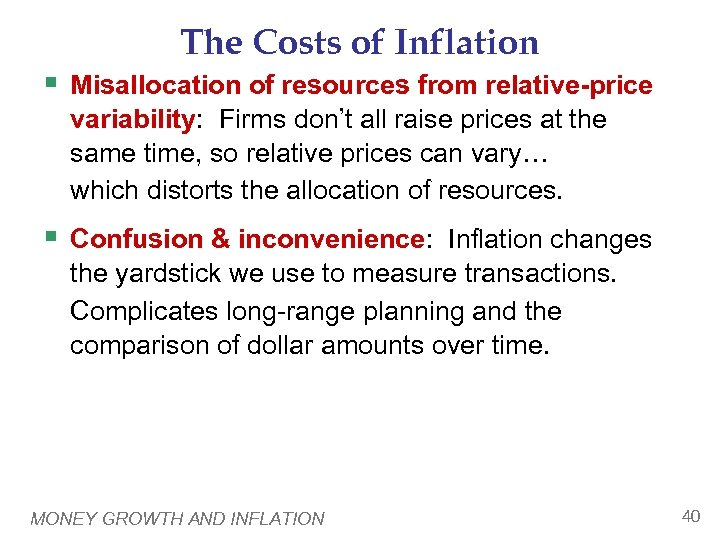The Costs of Inflation § Misallocation of resources from relative-price variability: Firms don’t all raise prices at the same time, so relative prices can vary… which distorts the allocation of resources. § Confusion & inconvenience: Inflation changes the yardstick we use to measure transactions. Complicates long-range planning and the comparison of dollar amounts over time. MONEY GROWTH AND INFLATION 40The Costs of Inflation § Tax distortions: Inflation makes nominal income grow faster than real income. Taxes are based on nominal income, and some are not adjusted for inflation. So, inflation causes people to pay more taxes even when their real incomes don’t increase. MONEY GROWTH AND INFLATION 41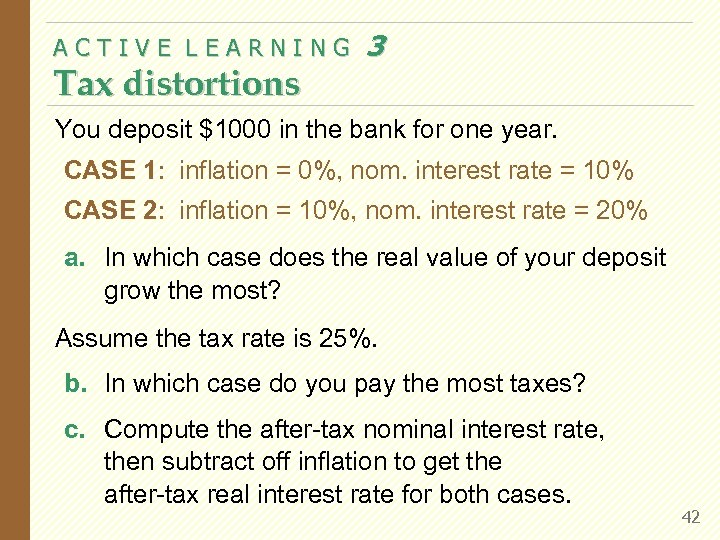ACTIVE LEARNING Tax distortions 3 You deposit \$1000 in the bank for one year. CASE 1: inflation = 0%, nom. interest rate = 10% CASE 2: inflation = 10%, nom. interest rate = 20% a. In which case does the real value of your deposit grow the most? Assume the tax rate is 25%. b. In which case do you pay the most taxes? c. Compute the after-tax nominal interest rate, then subtract off inflation to get the after-tax real interest rate for both cases. 42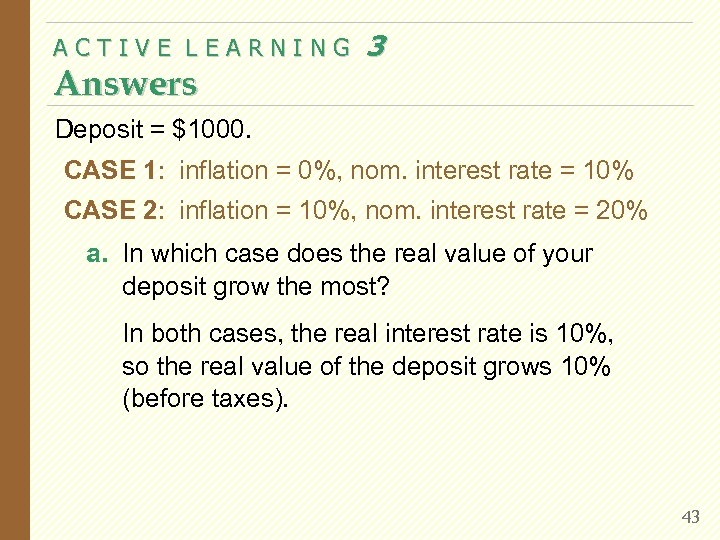ACTIVE LEARNING Answers 3 Deposit = \$1000. CASE 1: inflation = 0%, nom. interest rate = 10% CASE 2: inflation = 10%, nom. interest rate = 20% a. In which case does the real value of your deposit grow the most? In both cases, the real interest rate is 10%, so the real value of the deposit grows 10% (before taxes). 43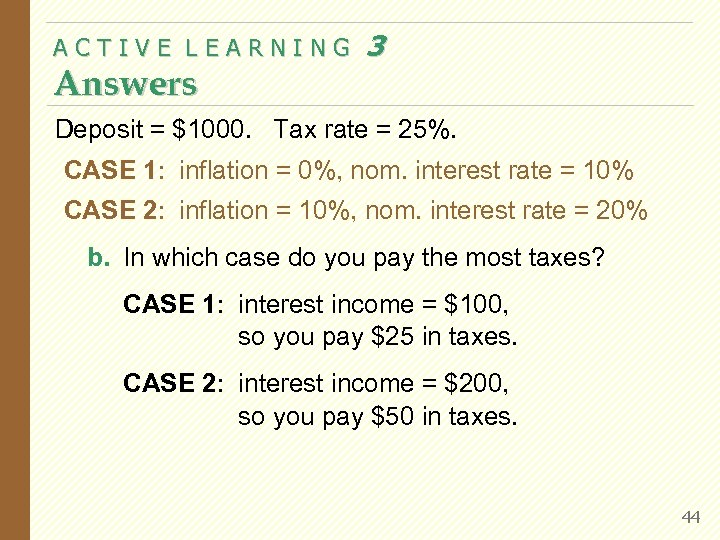ACTIVE LEARNING Answers 3 Deposit = \$1000. Tax rate = 25%. CASE 1: inflation = 0%, nom. interest rate = 10% CASE 2: inflation = 10%, nom. interest rate = 20% b. In which case do you pay the most taxes? CASE 1: interest income = \$100, so you pay \$25 in taxes. CASE 2: interest income = \$200, so you pay \$50 in taxes. 44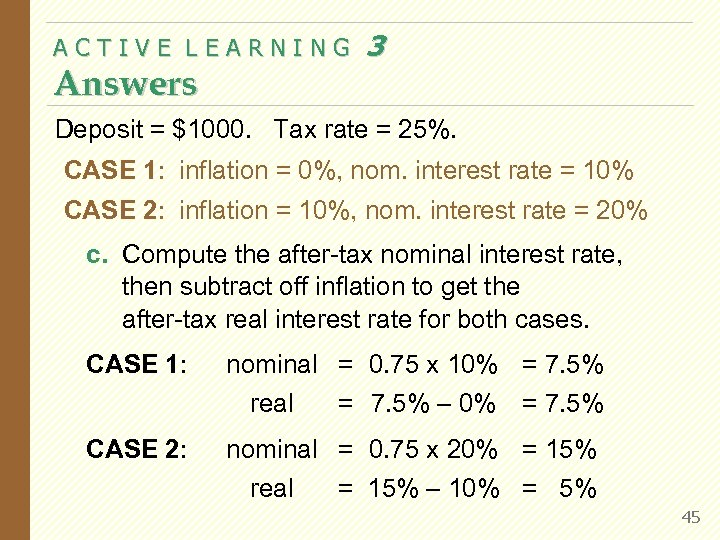ACTIVE LEARNING Answers 3 Deposit = \$1000. Tax rate = 25%. CASE 1: inflation = 0%, nom. interest rate = 10% CASE 2: inflation = 10%, nom. interest rate = 20% c. Compute the after-tax nominal interest rate, then subtract off inflation to get the after-tax real interest rate for both cases. CASE 1: nominal = 0. 75 x 10% = 7. 5% real CASE 2: = 7. 5% – 0% = 7. 5% nominal = 0. 75 x 20% = 15% real = 15% – 10% = 5% 45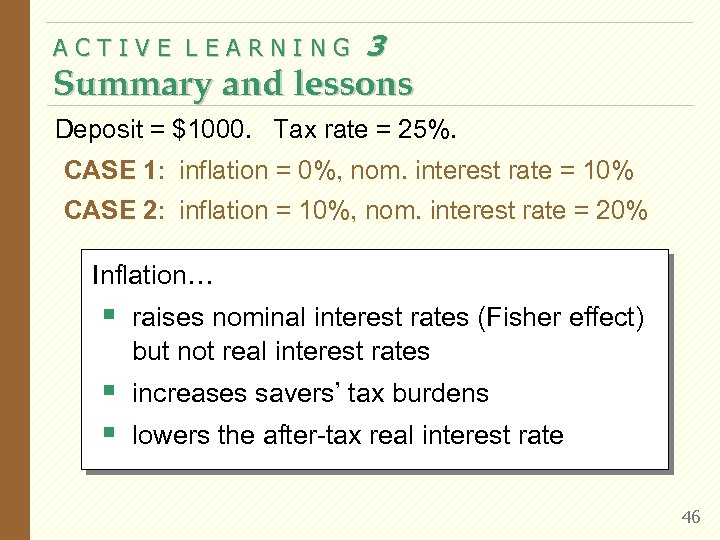ACTIVE LEARNING 3 Summary and lessons Deposit = \$1000. Tax rate = 25%. CASE 1: inflation = 0%, nom. interest rate = 10% CASE 2: inflation = 10%, nom. interest rate = 20% Inflation… § raises nominal interest rates (Fisher effect) but not real interest rates § increases savers’ tax burdens § lowers the after-tax real interest rate 46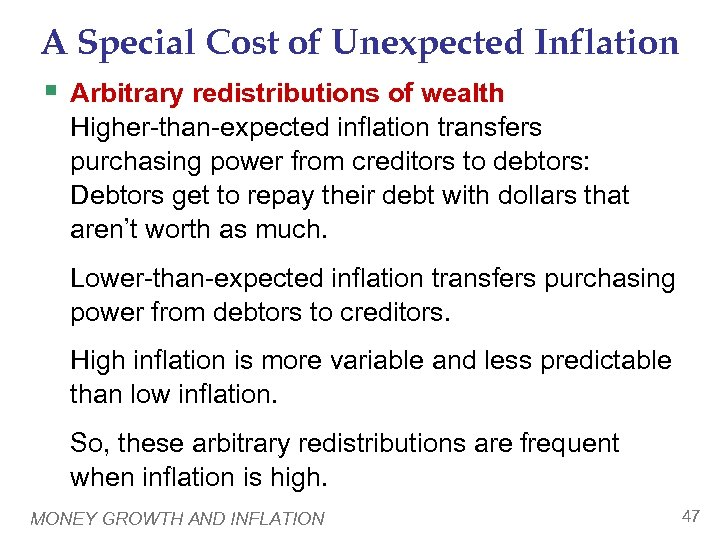A Special Cost of Unexpected Inflation § Arbitrary redistributions of wealth Higher-than-expected inflation transfers purchasing power from creditors to debtors: Debtors get to repay their debt with dollars that aren’t worth as much. Lower-than-expected inflation transfers purchasing power from debtors to creditors. High inflation is more variable and less predictable than low inflation. So, these arbitrary redistributions are frequent when inflation is high. MONEY GROWTH AND INFLATION 47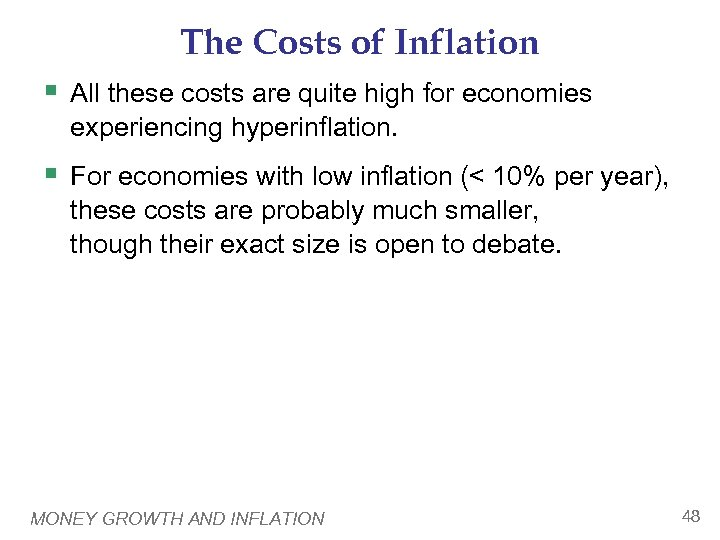The Costs of Inflation § All these costs are quite high for economies experiencing hyperinflation. § For economies with low inflation (< 10% per year), these costs are probably much smaller, though their exact size is open to debate. MONEY GROWTH AND INFLATION 48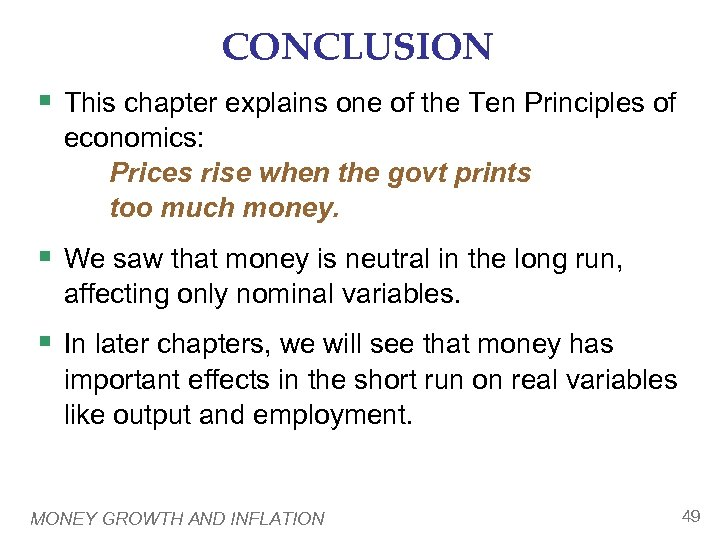CONCLUSION § This chapter explains one of the Ten Principles of economics: Prices rise when the govt prints too much money. § We saw that money is neutral in the long run, affecting only nominal variables. § In later chapters, we will see that money has important effects in the short run on real variables like output and employment. MONEY GROWTH AND INFLATION 49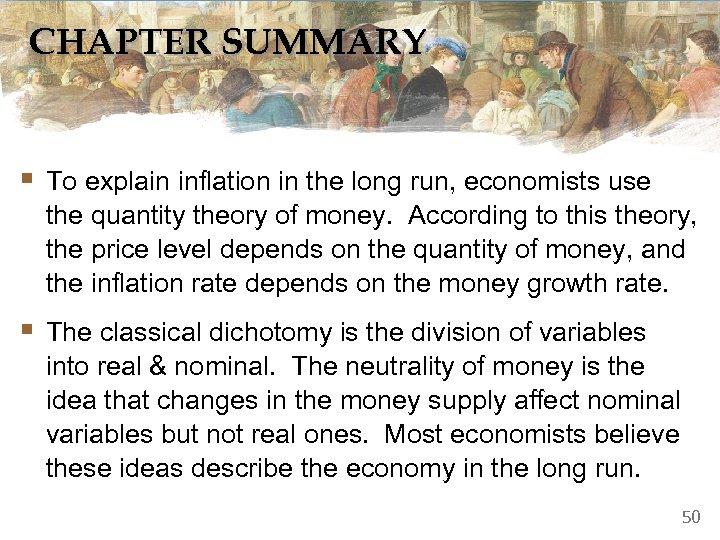CHAPTER SUMMARY § To explain inflation in the long run, economists use the quantity theory of money. According to this theory, the price level depends on the quantity of money, and the inflation rate depends on the money growth rate. § The classical dichotomy is the division of variables into real & nominal. The neutrality of money is the idea that changes in the money supply affect nominal variables but not real ones. Most economists believe these ideas describe the economy in the long run. 50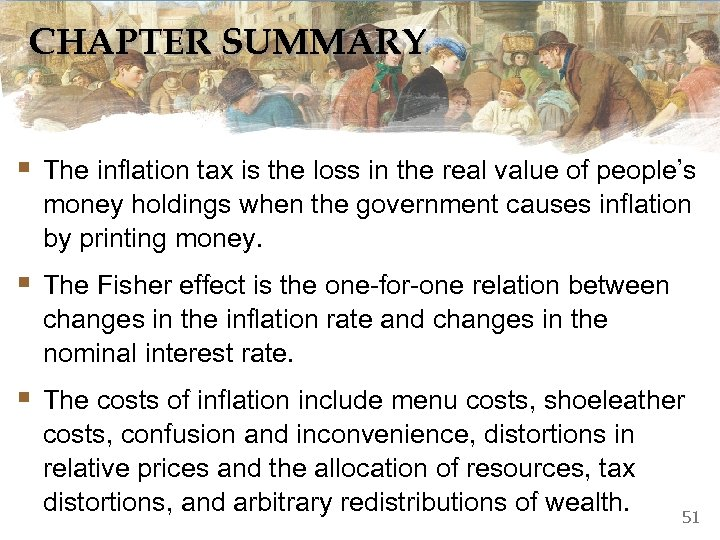CHAPTER SUMMARY § The inflation tax is the loss in the real value of people’s money holdings when the government causes inflation by printing money. § The Fisher effect is the one-for-one relation between changes in the inflation rate and changes in the nominal interest rate. § The costs of inflation include menu costs, shoeleather costs, confusion and inconvenience, distortions in relative prices and the allocation of resources, tax distortions, and arbitrary redistributions of wealth. 51